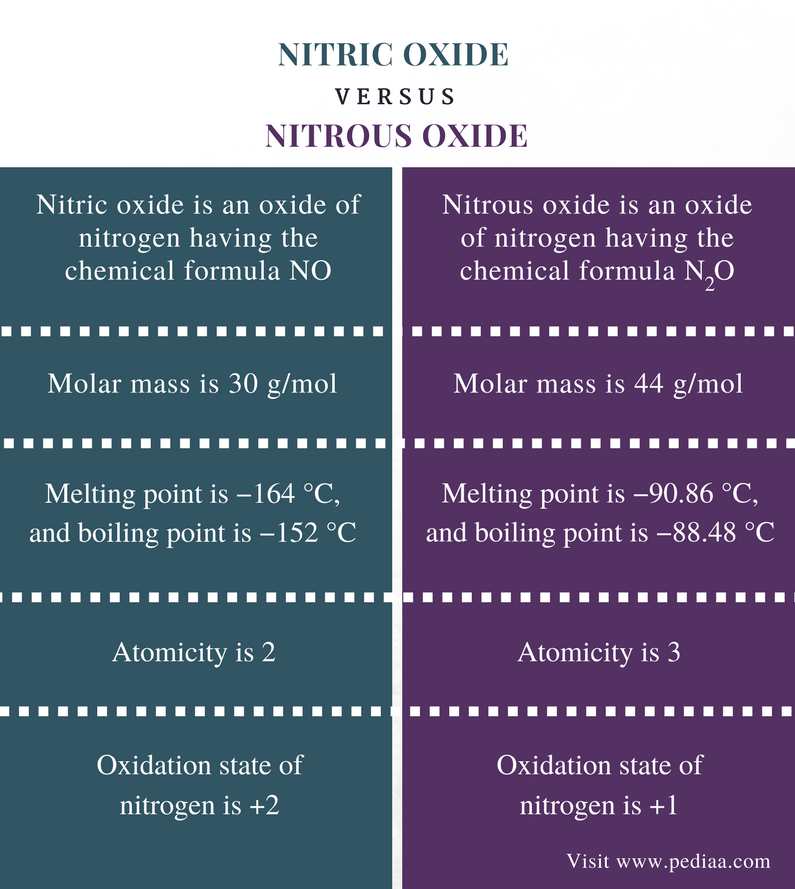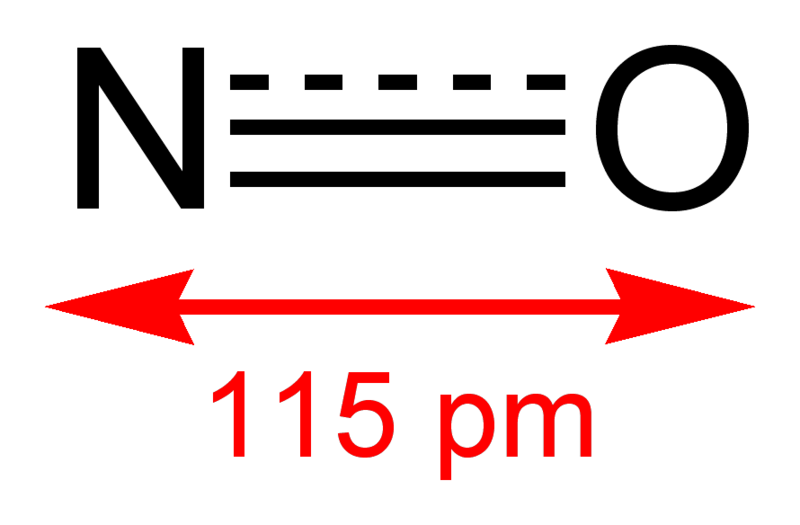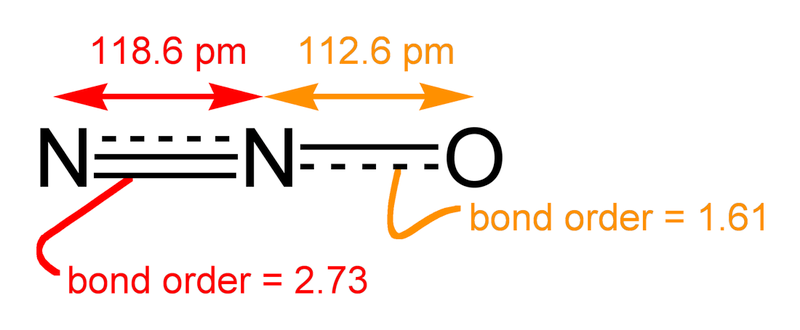# Difference Between Nitric Oxide and Nitrous Oxide

## Main Difference – Nitric Oxide vs Nitrous Oxide (Laughing Gas)

Nitric oxide and nitrous oxide are oxides of nitrogen. Nitrogen is a chemical element with the atomic number 7, and it is a nonmetal in the p block of the periodic table of elements. Nitrogen in its different oxides has different oxidation states. Oxidation state is the number of electrons a particular atom can lose, gain or share with another atom. In nitric oxide, nitrogen atom shares two electrons with an oxygen atom. Hence, the oxidation state of nitrogen in nitric oxide is +2. In contrast, the oxidation state of nitrogen in nitrous oxide is +1. This is the main difference between nitric oxide and nitrous oxide.

### Key Areas Covered

1. What is Nitric Oxide
– Definition, Chemical Properties, Reactions
2. What is Nitrous Oxide (Laughing Gas)
– Definition, Chemical Properties, Reactions
3. What are the Similarities Between Nitric Oxide and Nitrous Oxide
– Outline of Common Features
4. What is the Difference Between Nitric Oxide and Nitrous Oxide
– Comparison of Key Differences

Key Terms: Atomicity, Atomic Number, Dinitrogen Monoxide, Laughing Gas, Nitric Oxide, Nitrogen, Nitrous Oxide, Oxidation States, Oxygen## What is Nitric Oxide

Nitric oxide is an oxide of nitrogen having the chemical formula NO. Here, one nitrogen atom is bonded to an oxygen atom via covalent bonding. This means the nitrogen atom and the oxygen atom are sharing their unpaired electrons. In room temperature and pressure, nitric oxide is a toxic, colorless gas.Figure 1: Lewis Dot Structure for Nitric Oxide

The molar mass of this compound is 30 g/mol. The melting point of nitric oxide is −164 °C, and the boiling point is −152 °C. The nitric oxide molecule has a linear shape because there are only two atoms bonded to each other. When the unpaired electrons of nitrogen and oxygen are shared, there is another unpaired electron on the nitrogen atom. But there are no other unpaired electrons on oxygen atom to share. Then the unpaired electron is shared alone, among the two atoms. Therefore, the actual structure of the covalent bond is between a double bond and a triple bond. Then the bond length is 115 pm, which is a lower distance between the two atoms that the expected value.Figure 2: Actual Bond between Nitrogen and Oxygen in Nitric Oxide

Nitric oxide can form nitrogen dioxide in the presence of oxygen. But in water, nitric oxide reacts with oxygen and H2O to form nitrous acid (HNO2). When this gas is cooled, it forms nitric oxide dimers (N2O2). These are some major reactions of nitric oxide.

The oxidation state of nitrogen in nitric oxide is +2. That is because, the oxidation state of oxygen is -2 and since the nitrous oxide molecule is a neutral compound, the oxidation state of nitrogen should be +2.

## What is Nitrous Oxide (Laughing Gas)

Nitrous oxide is an oxide of nitrogen having the chemical formula N2O. The IUPAC name for nitrous oxide is dinitrogen monoxide. This gas is also called laughing gas because it causes insensibility to pain proceeded by mild hysteria, sometimes laughter.

The molar mass of this gas is 44 g/mol. It is a colorless gas at room temperature and pressure. The melting point of nitrous oxide is −90.86 °C, and the boiling point is −88.48 °C. The N2O molecule is composed of two nitrogen atoms and an oxygen atom. The nitrogen atoms are bonded to each other, and the oxygen atom is bonded to one of the two nitrogen atoms. When the chemical bonding between these atoms is considered, N2O shows resonance. There are two major resonance structures for this molecule.Figure 3: Resonance Structures of Nitrous Oxide

But the actual structure is a hybrid of those structures.Figure 4: Actual Structure of Nitrous Oxide

Nitrous oxide is considered as an inert gas at room temperature and pressure and has very few chemical reactions. But when the temperature is increased, its reactivity is also increased. For example, at 187 °C, nitrous oxide react with NaNH2 producing sodium azide (NaN3).

The oxidation state of nitrogen in nitrous oxide is +1. The oxidation state of oxygen is -2 and the nitrous oxide molecule is a neutral compound. Then oxidation state of both nitrogen atoms should be +2 in order to balance the equation.

Oxidation state of nitrous oxide = 0

Oxidation state of oxygen atom = -2

Therefore,

N2O = 2(N) + (O)
0       = 2(N) + (-2)
2(N) = +2
(N)    = +1

## Similarities Between Nitric Oxide and Nitrous Oxide

• Both are colorless gases at room temperature and pressure.
• Both are composed of nitrogen and oxygen atoms.
• Both are covalent compounds.

## Difference Between Nitric Oxide and Nitrous Oxide

### Definition

Nitric Oxide: Nitric oxide is an oxide of nitrogen having the chemical formula NO.

Nitrous Oxide: Nitrous oxide is an oxide of nitrogen having the chemical formula N2O.

### Molar Mass

Nitric Oxide: The molar mass of nitric oxide is 30 g/mol.

Nitrous Oxide: The molar mass of nitrous oxide is 44 g/mol.

### Melting Point and Boiling Point

Nitric Oxide: The melting point of nitric oxide is −164 °C, and the boiling point is −152 °C.

Nitrous Oxide: The melting point of nitrous oxide is −90.86 °C, and the boiling point is −88.48 °C.

### Atomicity

Nitric Oxide: The atomicity of nitric oxide is 2.

Nitrous Oxide: The atomicity of nitrous oxide is 3.

### Oxidation State of Nitrogen

Nitric Oxide: The oxidation state of nitrogen in nitric oxide is +2.

Nitrous Oxide: The oxidation state of nitrogen in nitrous oxide is +1.

### Conclusion

Nitrogen is a nonmetallic chemical element in the group 5 of the periodic table. It can form many oxide compounds. Nitric oxide and nitrous oxide are such two compounds. The main difference between nitric oxide and nitrous oxide is that the nitrogen of nitric oxide has the oxidation state +2 whereas the oxidation state of nitrogen in nitrous oxide is +1.

##### References:

1. “Nitric oxide.” Encyclopædia Britannica, Encyclopædia Britannica, inc., 25 Aug. 2017, Available here.
2. “Nitrous oxide.” Wikipedia, Wikimedia Foundation, 17 Dec. 2017, Available here.

##### Image Courtesy:

1. “Nitric oxide Lewis” By Bioika0201 – Own work (CC BY-SA 4.0) via Commons Wikimedia
2. “Nitric-oxide-2D” (Public Domain)  via Commons Wikimedia
3. “Nitrous-oxide-2D-VB” By WhiteTimberwolf, Ben Mills (PNG version) – Own work (Public Domain) via Commons Wikimedia
4. “Nitrous-oxide-2D-dimensions” (Public Domain) via Commons Wikimedia